﻿ How to Calculate VCE of a Transistor# How to Calculate VCE of a Transistor

VCE, the voltage that falls across the collector-emitter junction of a bipolar junction transistor, is a crucial voltage to DC analysis of a transistor circuit because it is the voltage that determines the load line and Q-point of a transistor.

VCE is calculated by the formula below: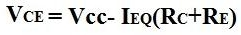Example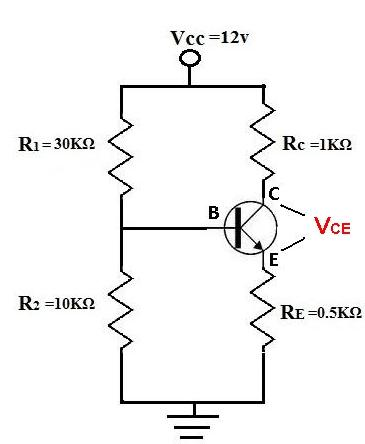In order to calculate VCE, we must first calculate IEQ: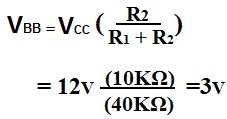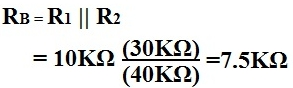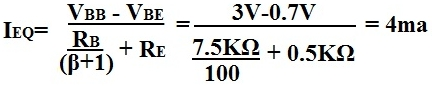Now that we have IEQ, we can solve for VCE:Related Resources

What is Open Collector Output?